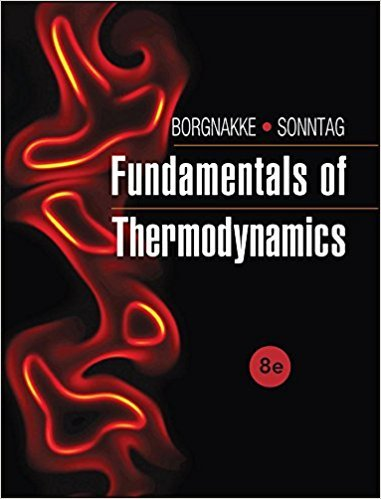×
Get Full Access to Fundamentals Of Thermodynamcs - 8 Edition - Chapter 5 - Problem 108hp
Get Full Access to Fundamentals Of Thermodynamcs - 8 Edition - Chapter 5 - Problem 108hp

×

# A farmer runs a heat pump with a motor of 2 kW. It shouldISBN: 9781118131992 58

## Solution for problem 108HP Chapter 5

Fundamentals of Thermodynamcs | 8th Edition

• Textbook Solutions
• 2901 Step-by-step solutions solved by professors and subject experts
• Get 24/7 help from StudySoup virtual teaching assistantsFundamentals of Thermodynamcs | 8th Edition

4 5 1 389 Reviews
23
1
Problem 108HP

Problem 108HP

A farmer runs a heat pump with a motor of 2 kW. It should keep a chicken hatchery at 30°C; the hatchery loses energy at a rate of 0.5 kW per degree difference to the colder ambient. The heat pump has a COP that is 50% that of a Carnot heat pump. What is the minimum ambient temperature for which the heat pump is sufficient?

Step-by-Step Solution:
Step 1 of 3

Spencer Kociba CHEM 101 Lecture 11/15/16 THERMOCHEMISTRY ● Enthalpy ○ Represented as ΔH ○ Internal energy + pressure*volume ■ We will mostly be concerned with constant pressure. This is where the formula ΔH = ΔE + PΔV comes from ○ ΔH = q (enthalpy=heat gained or lost in the system) ● Properties of Enthalpy (ΔH ) ○ ΔH is directly proportional to mass ○ ΔH for a reverse reaction is equal in magnitude (quantity) but opposite in sign for the forwards reaction ○ Hess’s Law: ΔH is independent of the number of steps involved ■ If Reaction(1) + Reaction(2)=Reaction(3), then ΔH =3ΔH + 1H 2 ● Endothermic reactions ○ Gains heat from sur

Step 2 of 3

Step 3 of 3

##### ISBN: 9781118131992

The full step-by-step solution to problem: 108HP from chapter: 5 was answered by , our top Engineering and Tech solution expert on 08/03/17, 05:05AM. The answer to “A farmer runs a heat pump with a motor of 2 kW. It should keep a chicken hatchery at 30°C; the hatchery loses energy at a rate of 0.5 kW per degree difference to the colder ambient. The heat pump has a COP that is 50% that of a Carnot heat pump. What is the minimum ambient temperature for which the heat pump is sufficient?” is broken down into a number of easy to follow steps, and 65 words. This full solution covers the following key subjects: heat, Pump, ambient, hatchery, loses. This expansive textbook survival guide covers 7 chapters, and 1462 solutions. Fundamentals of Thermodynamcs was written by and is associated to the ISBN: 9781118131992. This textbook survival guide was created for the textbook: Fundamentals of Thermodynamcs , edition: 8. Since the solution to 108HP from 5 chapter was answered, more than 277 students have viewed the full step-by-step answer.

Unlock Textbook Solution